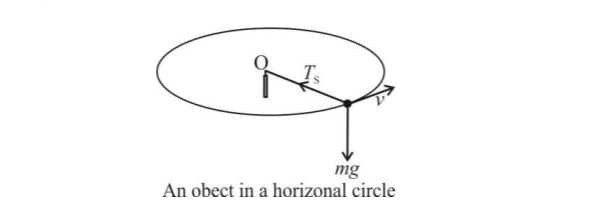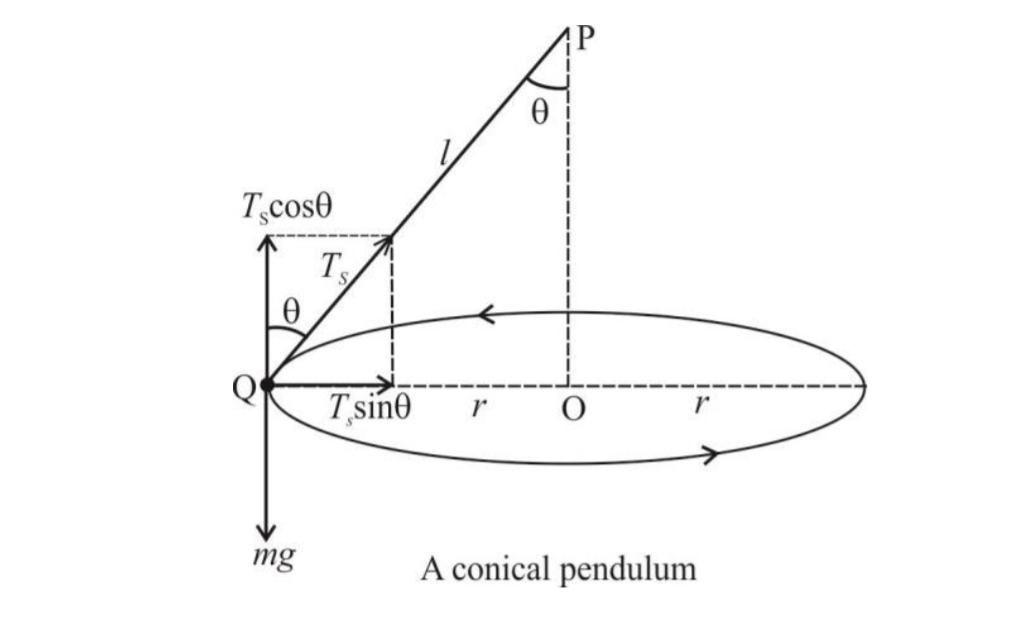# Motion in Horizontal CircleMotion in a horizontal circle↪ Suppose an object of mass m tied at one end of a string is whirling in a horizontal circle of radius r and center O.
↪ The object is moving with a uniform linear velocity v and angular velocity ω.
↪ Since the weight mg of the object acts at right angles to the tension TS in the string, the force toward the center O is↪ Thus the tension in the string is equal to the centripetal force.

⁕ Conical Pendulum↪ A conical pendulum is a revolving object suspended by a string from a rigid support about which the string makes a right circular cone of semi-angle θ with the vertical.
↪ Suppose a small object of mass m is tied at one end of a string of length l and is then whirling in a horizontal circle of radius r with center O which is exactly below a fixed point P.
↪ The object moves with constant linear speed v and angular speed ω in the circle.
This is a conical pendulum.

↪ Let TS be the tension in the string and let θ be the angle made by the string with the vertical and hence the angle between TS and the vertical.
↪ The component of TS along the vertical is balanced by the weight mg of the object.
That is,and the component of TS along the horizontal or toward the center of the circle provides the necessary centripetal force.
That is,Dividing (2) by (1), we getFrom right ∆OPQ,
r = lsinθ … (5)
The angular velocity ω of the object is↪ Therefore the period T of revolution is↪ This is the period of revolution of the conical pendulum.

From (1), we have